# RRB ALP 2018 Practice Test Papers | Reasoning Questions (Day-29)

Dear Aspirants, Here we have given the Important RRB ALP & Technicians Exam 2018 Practice Test Papers. Candidates those who are preparing for RRB ALP 2018 can practice these Reasoning Questions to get more confidence to Crack RRB 2018 Examination.

[WpProQuiz 1961]

Click “Start Quiz” to attend these Questions and view Solutions

1. If 4 × 2 × 6 = 1626, 3 × 7 × 4 = 974, then 5 × 6 × 8 =?

(a) 3658

(b) 2568

(c) 5664

(d) 6456

1. Which one set of letters when sequentially placed at the gaps in the given letter series shall complete it? a _ b _ c _ a _ bc _ b _ cb

(a) acbcab

(b) ccbcca

(c) ccaccb

(d) cacabc

1. If PAINT is coded as 74128 and EXCEL is coded as 93596, how is ACCEPT coded?

(a) 459578

(b) 457958

(c) 459758

(d) 455978

1. Arrange the following words in their descending order:
2. Weekly 2. Bi-annual 3. Fortnightly 4. Monthly 5. Annual

(a) 1, 3, 4, 2, 5

(b) 2, 5, 4, 1, 3

(c) 4, 1, 2, 3, 5

(d) 5, 2, 4, 3, 1

1. Pointing to a man, a lady said “His mother is the only daughter of my mother”. How is the lady related to the man?

(a) Mother

(b) Daughter

(c) Sister

(d) Aunt

1. If 63 – 30 = 30, 72 – 10 = 40, then 81 – 60 =?

(a) 50

(b) 35

(c) 15

(d) 20

1. Select the related letters from the given alternatives. YWUS: QOMK:: ZXVT:?

(a) YWUS

(b) RPNL

(c) RPNM

(d) XWUT

1. Select the missing number from the given responses. 1, 3, 7, 15, 31, 63, 127,?.

(a) 260

(b) 275

(c) 350

(d) 255

1. Put the correct mathematical signs in the following equation from the given alternatives. 33? 11? 3? 6 = 115

(a) ×, ÷, –

(b) ÷, ×, ×

(c) –, ×, +

(d) +, –, ×

1. 32, 58, 92, 134,?.

(a) 169

(b) 184

(c) 194

(d) 156

4 × 2 × 6 = 1626 = (42 )26 = 1626

3 × 7 × 4 = 974 = (32 )74 = 974

5 × 6 × 8 = (52 )68 = 2568

acb c cb acb ccb acb

1. Answer: (d)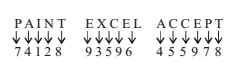Annual – 1 year

Monthly – 30 days

Weekly – 7 days

Biannual – 6 month

Fortnightly – 15 days.

1. Answer: (a)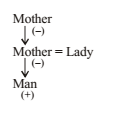(6 + 3) – (3 + 0) = 6 × 5 = 30

(7 + 2) – (1 + 0) = 8 × 5 = 40

(8 + 1) – (6 + 0) = 3 × 5 = 15

1. Answer: (b)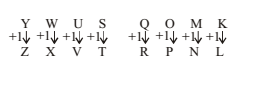2. Answer: (d)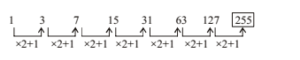33 × 11 ÷ 3 – 6 = 115

363 /3-6= 121 – 6 = 115

1. Answer: (b)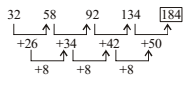RRB ALP 2018 Practice Test Papers | Reasoning Questions (Day-1)

RRB ALP 2018 Practice Test Papers | Reasoning Questions (Day-2)

RRB ALP 2018 Practice Test Papers | Reasoning Questions (Day-3)

RRB ALP 2018 Practice Test Papers | Reasoning Questions (Day-4)

RRB ALP 2018 Practice Test Papers | Reasoning Questions (Day-5)

RRB ALP 2018 Practice Test Papers | Reasoning Questions (Day-6)

RRB ALP 2018 Practice Test Papers | Reasoning Questions (Day-7)

RRB ALP 2018 Practice Test Papers | Reasoning Questions (Day-8)

RRB ALP 2018 Practice Test Papers | Reasoning Questions (Day-9)

RRB ALP 2018 Practice Test Papers | Reasoning Questions (Day-10)

RRB ALP 2018 Practice Test Papers | Reasoning Questions (Day-11)

RRB ALP 2018 Practice Test Papers | Reasoning Questions (Day-12)

RRB ALP 2018 Practice Test Papers | Reasoning Questions (Day-13)

RRB ALP 2018 Practice Test Papers | Reasoning Questions (Day-14)

RRB ALP 2018 Practice Test Papers | Reasoning Questions (Day-15)

RRB ALP 2018 Practice Test Papers | Reasoning Questions (Day-16)

RRB ALP 2018 Practice Test Papers | Reasoning Questions (Day-17)

RRB ALP 2018 Practice Test Papers | Reasoning Questions (Day-18)

RRB ALP 2018 Practice Test Papers | Reasoning Questions (Day-19)

RRB ALP 2018 Practice Test Papers | Reasoning Questions (Day-20)

RRB ALP 2018 Practice Test Papers | Reasoning Questions (Day-21)

RRB ALP 2018 Practice Test Papers | Reasoning Questions (Day-22)

RRB ALP 2018 Practice Test Papers | Reasoning Questions (Day-23)

RRB ALP 2018 Practice Test Papers | Reasoning Questions (Day-24)

RRB ALP 2018 Practice Test Papers | Reasoning Questions (Day-25)

RRB ALP 2018 Practice Test Papers | Reasoning Questions (Day-26)

RRB ALP 2018 Practice Test Papers | Reasoning Questions (Day-27)

RRB ALP 2018 Practice Test Papers | Reasoning Questions (Day-28)